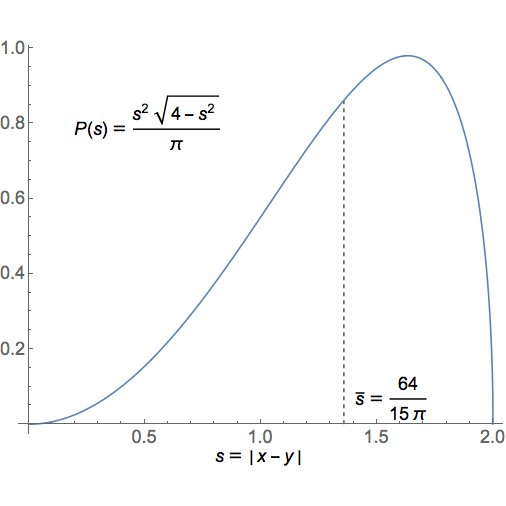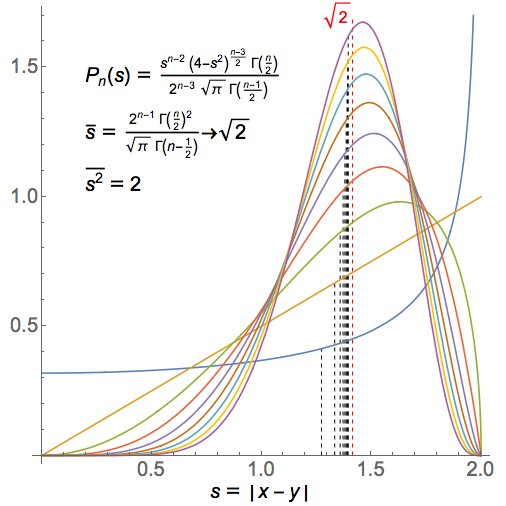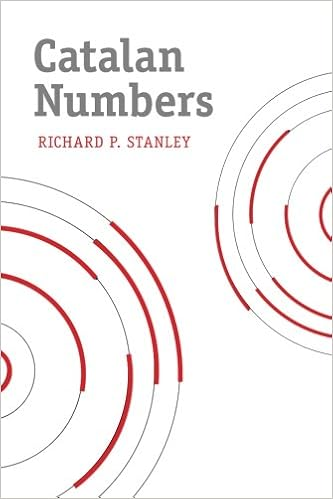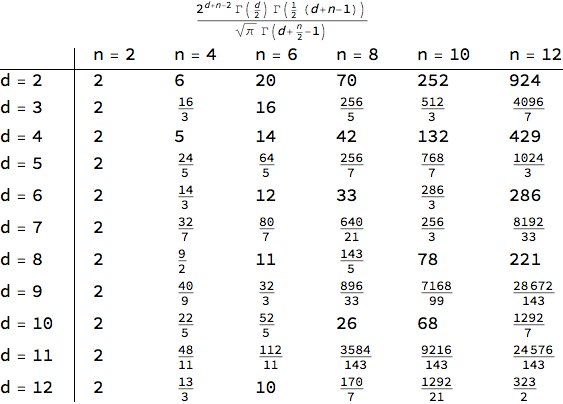#### Howdy, Stranger!

It looks like you're new here. If you want to get involved, click one of these buttons!

Options

# Just For Fun 4

edited July 2018 in Chat

Recently Greg Egan and I were talking about quaternions, and I asked him to compute the mean distance between two randomly chosen unit quaternions.

You don't need to know anything about quaternions to do this. Another way to phrase the question is: what's the average distance between two randomly chosen points of the unit sphere in $$\mathbb{R}^4$$?

Yet another way to put it is this: if you sit at the north pole of this sphere, and randomly pick another point on the sphere, what is the expected value of its distance from you?"

(Here and in all that follows, distances are measured using straight lines in $$\mathbb{R}^n$$, not using geodesics on the sphere. There is a unique rotation-invariant probability measure on a sphere of any dimension, and that's what we use to randomly choose a point on a sphere.)

Greg computed the answer, which is $$64/15\pi \approx 1.35812218105$$. But he went further and computed the whole probability distribution:I tried to figure out why the answer looked like this. I noticed, that if if you take a unit sphere in any dimension, and you sit at the north pole, the points on the equator are at distance $$\sqrt{2} \approx 1.41421356$$. This is pretty close to $$1.35812218105$$.

I wrote:

Neat! I'm kind of surprised the mode doesn't occur at $\sqrt(2)$, because if you're at the north pole it'll seem like "most" points are at the equator. Maybe I'm making an elementary mistake, confusing parametrization by angle and by distance.

That was indeed the problem.

But as you go to higher dimensions, points near the equator should become more and more probable (you can see this using a little calculation). So, I predicted that if we repeat this calculation for spheres of different dimensions, the probability distribution should spike more and more strongly near $$\sqrt{2}$$.

• Options
1.

Egan showed that this was indeed true:He wrote:

Here’s the general formula for the distribution, with plots for n=2,...,10. The mean distance does tend to √2, and the mean of the squared distance is always exactly 2, so the variance tends to zero.

Comment Source:<a href = "https://twitter.com/gregeganSF/status/1015372602255163392">Egan showed</a> that this was indeed true: <center><img src = "http://math.ucr.edu/home/baez/mathematical/distance_between_points_on_unit_sphere_histograms_egan.jpg"></center> He wrote: > Here’s the general formula for the distribution, with plots for n=2,...,10. The mean distance does tend to √2, and the mean of the squared distance is always exactly 2, so the variance tends to zero. 
• Options
2.
edited July 2018

Then Dan Piponi, another one of my internet math pals, went back to the 4d case and thought about the moments of the distance for 2 randomly chosen points on the unit sphere in $$\mathbb{R}^4$$. The first moment is the mean, the second moment is the variance, and so on.

He notice that the even moments were the Catalan numbers

$$2, 5, 14, 42, 132 \dots$$

Pick a pair of points uniformly randomly on the 4-sphere. Let S be the distance between them. E[S²ⁿ] is the (n+1)-th Catalan number.

This was funny, because he'd just managed to buy a hardback copy of Richard P. Stanley's book on Catalan numbers on Amazon for just 11 dollars, thanks to some glitch that quickly went away.Comment Source:Then [Dan Piponi](https://twitter.com/sigfpe/status/1015384915058176001), another one of my internet math pals, went back to the 4d case and thought about the _moments_ of the distance for 2 randomly chosen points on the unit sphere in \$$\mathbb{R}^4\$$. The first moment is the mean, the second moment is the variance, and so on. He notice that the _even_ moments were the [Catalan numbers](https://en.wikipedia.org/wiki/Catalan_number) $$2, 5, 14, 42, 132 \dots$$ > Pick a pair of points uniformly randomly on the 4-sphere. Let S be the distance between them. E[S²ⁿ] is the (n+1)-th Catalan number. This was funny, because he'd just managed to buy a hardback copy of Richard P. Stanley's book on Catalan numbers on Amazon for just 11 dollars, thanks to some glitch that quickly went away. <center><img src = "https://images-na.ssl-images-amazon.com/images/I/41-2V4T-d3L._SX331_BO1,204,203,200_.jpg"></center>
• Options
3.
edited July 2018

I asked if we got other interesting sequences of numbers by looking at the moments for the distance of two randomly chosen points on the sphere in other dimensions.

Greg Egan, with his usual unstoppable force, worked out a chart of the $$n$$th moment of the distance of two randomly chosen points on the unit sphere in $$\mathbb{R}^d$$, at least when $$n$$ is even:He wrote:

These are the nth moments for even n, for dimensions 2 to 12. So generally these are not all integers.

You can see the Catalan numbers on the $$d = 4$$ row. But what else do you see?

Comment Source:I asked if we got other interesting sequences of numbers by looking at the moments for the distance of two randomly chosen points on the sphere in other dimensions. [Greg Egan, with his usual unstoppable force](https://twitter.com/gregeganSF/status/1015393738003132417), worked out a chart of the \$$n\$$th moment of the distance of two randomly chosen points on the unit sphere in \$$\mathbb{R}^d\$$, at least when \$$n\$$ is even: <center><img src = "http://math.ucr.edu/home/baez/mathematical/distance_between_points_on_unit_sphere_moments_egan.jpg"></center> He wrote: > These are the nth moments for even n, for dimensions 2 to 12. So generally these are not all integers. You can see the Catalan numbers on the \$$d = 4\$$ row. But what else do you see?
• Options
4.

Dan Piponi spotted an amazing pattern:

You left out the row for d=1. It's a row of integers too. So the dimensions that give associative division algebras give integers. I bet there's an algebraic argument that works only for those cases.

Amazing! The unit spheres in $$d = 1, 2,$$ and $$4$$ dimensions are the only spheres that are also groups. They are unit real numbers, the unit complex numbers and the unit quaternions.

For some reason the even moments of the distance of two randomly chosen points on the sphere are integers in these three cases! Why?

Comment Source:Dan Piponi spotted an amazing pattern: > You left out the row for d=1. It's a row of integers too. So the dimensions that give associative division algebras give integers. I bet there's an algebraic argument that works only for those cases. Amazing! The unit spheres in \$$d = 1, 2, \$$ and \$$4\$$ dimensions are the only spheres that are also _groups_. They are unit real numbers, the unit complex numbers and the unit quaternions. For some reason the even moments of the distance of two randomly chosen points on the sphere are _integers_ in these three cases! Why? 
• Options
5.
edited July 2018

I had to figure it out... and I think I did. It took me a few tweets to fumble my way to the answer.

A vague idea:

There must be a purely gamma-function-theoretic way to understand this integrality, but it would be really fun to have an argument based on harmonic analysis on the spheres (decomposing the distance function into harmonics, say), connecting it to division algebras.

A better idea:

For example, for the spheres that are groups ($$S^0, S^1$$ and $$S^3$$) we can use Peter-Weyl to decompose $$L^2(S^n)$$ as a sum of reps $$R \otimes R^\ast$$ where $$R$$ ranges over all irreps of that group. The powers of the distance function live in there.

A better idea:

Oh - it's the square of the distance function that's a polynomial on $$\mathbb{R}^n$$, and thus has a manageable expression in terms of spherical harmonics when we restrict it to the sphere. This kinda explains why even moments of the distance function are nicer.

An actual inspiration:

I bet the in the cases of spheres that are groups, the $$2n$$th integer Greg computed above is the dimension of the trivial part of the $$n$$th tensor power of a certain rep of that group!

A concrete plan of attack:

I conjecture the square of the distance function is the character of a certain rep $$X$$. This implies that the $$2n$$th power of the distance function is the character of $$X^{\otimes n}$$. The integral of this function over the sphere (the group) is then the dim of the trivial subrep.

And thus it must be an integer! - if my conjecture is true. But the conjecture can be checked on a case-by-case basis, since we just need to understand the reps of $$S^0 = \mathbb{Z}/2, S^1 = \mathrm{U}(1)$$ and $$S^3 = \mathrm{SU}(2)$$, and express the distance-squared function as a character of some rep.

A correction:

Hmm, it's not quite that simple. For $$S^0 \subset \mathbb{R}$$ the distance-squared function is $$0$$ at the identity $$+1$$ and $$4$$ at -1. This isn't a character of a rep, since it vanishes at the identity. But it's a difference of characters of reps - or a character of a 'virtual rep'. Probably enough.

The rest of the details should be straightforward.

Comment Source:I had to figure it out... and I think I did. It took me a few tweets to fumble my way to the answer. A vague idea: > There must be a purely gamma-function-theoretic way to understand this integrality, but it would be really fun to have an argument based on harmonic analysis on the spheres (decomposing the distance function into harmonics, say), connecting it to division algebras. A better idea: > For example, for the spheres that are groups (\$$S^0, S^1\$$ and \$$S^3\$$) we can use Peter-Weyl to decompose \$$L^2(S^n)\$$ as a sum of reps \$$R \otimes R^\ast\$$ where \$$R\$$ ranges over all irreps of that group. The powers of the distance function live in there. A better idea: > Oh - it's the *square* of the distance function that's a polynomial on \$$\mathbb{R}^n\$$, and thus has a manageable expression in terms of spherical harmonics when we restrict it to the sphere. This kinda explains why even moments of the distance function are nicer. An actual inspiration: > I bet the in the cases of spheres that are groups, the \$$2n\$$th integer Greg computed above is the dimension of the trivial part of the \$$n\$$th tensor power of a certain rep of that group! A concrete plan of attack: > I conjecture the square of the distance function is the character of a certain rep \$$X\$$. This implies that the \$$2n\$$th power of the distance function is the character of \$$X^{\otimes n}\$$. The integral of this function over the sphere (the group) is then the dim of the trivial subrep. > And thus it must be an integer! - if my conjecture is true. But the conjecture can be checked on a case-by-case basis, since we just need to understand the reps of \$$S^0 = \mathbb{Z}/2, S^1 = \mathrm{U}(1)\$$ and \$$S^3 = \mathrm{SU}(2)\$$, and express the distance-squared function as a character of some rep. A correction: > Hmm, it's not quite that simple. For \$$S^0 \subset \mathbb{R}\$$ the distance-squared function is \$$0\$$ at the identity \$$+1\$$ and \$$4\$$ at -1. This isn't a character of a rep, since it vanishes at the identity. But it's a difference of characters of reps - or a character of a 'virtual rep'. Probably enough. The rest of the details should be straightforward.
• Options
6.
edited July 2018

Egan, meanwhile, got a simple formula for the $$n$$th moment in the $$d = 2$$ case, at least when $$n$$ is even:

As well as: moment(4,n) = Catalan(n/2+1) we have: moment(2,n) = $$n \choose {n/2}$$.

That gives these numbers:

$$2, 6, 10, 70 , 252, \dots$$ Straight down the middle of Pascal's triangle!Comment Source:Egan, meanwhile, got a simple formula for the \$$n\$$th moment in the \$$d = 2\$$ case, at least when \$$n\$$ is even: > As well as: moment(4,n) = Catalan(n/2+1) we have: moment(2,n) = \$$n \choose {n/2}\$$. That gives these numbers: $$2, 6, 10, 70 , 252, \dots$$ Straight down the middle of Pascal's triangle! <center><img src = "https://upload.wikimedia.org/wikipedia/commons/thumb/0/03/Pascal%27s_Triangle_rows_0-16.svg/640px-Pascal%27s_Triangle_rows_0-16.svg.png"></center> 
• Options
7.
edited July 2018

It appears that

$$\mathrm{moment}(3,n) = \frac{2^n}{\frac{n}{2} + 1}$$ Other than that I can't make out any other patterns, but hopefully I can find something later...

Comment Source:It appears that $\mathrm{moment}(3,n) = \frac{2^n}{\frac{n}{2} + 1}$ Other than that I can't make out any other patterns, but hopefully I can find something later...
• Options
8.
edited July 2018

Thanks, Matthew! I want to take Greg Egan's formula and convert it from gamma functions to factorials. Let me at least take the first step: converting it to LaTeX:

$$\text{moment}(d,n) = \frac{2^{d+n-2} \Gamma(\frac{d}{2}) \Gamma(\frac{1}{2} (d+n-1))}{\sqrt{\pi} \, \Gamma(d+ \frac{n}{2} - 1)}$$

Comment Source:Thanks, Matthew! I want to take Greg Egan's formula and convert it from gamma functions to factorials. Let me at least take the first step: converting it to LaTeX: $\text{moment}(d,n) = \frac{2^{d+n-2} \Gamma(\frac{d}{2}) \Gamma(\frac{1}{2} (d+n-1))}{\sqrt{\pi} \, \Gamma(d+ \frac{n}{2} - 1)}$
• Options
9.
edited July 2018

Now, the above formula is mildly annoying because if $$k$$ is an integer

$$\Gamma(k) = (k-1)!$$ (where, by the way, the "-1" offers absolutely no advantage: it's a completely stupid convention), while

$$\Gamma(k + \frac{1}{2}) = {(2k)! \over 4^k k!} \sqrt{\pi} = \frac{(2k-1)!!}{2^k} \sqrt{\pi}$$ so

$$\text{moment}(d,n) = \frac{2^{d+n-2} \Gamma(\frac{d}{2}) \Gamma(\frac{1}{2} (d+n-1))}{\sqrt{\pi} \, \Gamma(d+ \frac{n}{2} - 1)}$$ seems to involve 4 cases, depending on the parity of both $$d$$ and $$n$$.

It makes plenty of sense to only focus on the even moments, $$n$$ even, say $$n = 2m$$, and then

$$\text{moment}(d,2m) = \frac{2^{d+2m-2} \Gamma(\frac{d}{2}) \Gamma(m+ \frac{1}{2} (d-1))}{\sqrt{\pi} \, \Gamma(d+ m - 1)}$$ but we still have two cases, $$d$$ even and $$d$$ odd.

I'm more interested in the case $$d$$ even because Greg Egan has done computer calculations suggesting that in these cases, only finitely many primes show up as denominators of the moments $$\text{moment}(d,2m)$$ written in lowest terms:

I tallied all the denominators for even n=2,...,100,000:

d=6: {1,49234},{3,766}

d=8: {1,49017},{2,13},{5,970}

d=10: {1,47149},{5,1893},{7,911},{35,47}

d=12: {1,46505},{2,10},{3,1211},{7,1823},{9,358},{14,2},{21,67},{63,24}

For odd d, the denominators quickly get large and various.

I think the first number in these ordered pairs is the denominator while the second is how many times it shows up in $$\text{moment}(d,n)$$ for even $$n$$ between 2 and 100,000.

Comment Source:Now, the above formula is mildly annoying because if \$$k\$$ is an integer $\Gamma(k) = (k-1)!$ (where, by the way, the "-1" offers absolutely no advantage: it's a completely stupid convention), while $\Gamma(k + \frac{1}{2}) = {(2k)! \over 4^k k!} \sqrt{\pi} = \frac{(2k-1)!!}{2^k} \sqrt{\pi}$ so $\text{moment}(d,n) = \frac{2^{d+n-2} \Gamma(\frac{d}{2}) \Gamma(\frac{1}{2} (d+n-1))}{\sqrt{\pi} \, \Gamma(d+ \frac{n}{2} - 1)}$ seems to involve 4 cases, depending on the parity of both \$$d\$$ and \$$n\$$. It makes plenty of sense to only focus on the _even_ moments, \$$n\$$ even, say \$$n = 2m\$$, and then $\text{moment}(d,2m) = \frac{2^{d+2m-2} \Gamma(\frac{d}{2}) \Gamma(m+ \frac{1}{2} (d-1))}{\sqrt{\pi} \, \Gamma(d+ m - 1)}$ but we still have two cases, \$$d\$$ even and \$$d\$$ odd. I'm more interested in the case \$$d\$$ even because Greg Egan has done computer calculations suggesting that in these cases, _only finitely many primes_ show up as denominators of the moments \$$\text{moment}(d,2m) \$$ written in lowest terms: > I tallied all the denominators for even n=2,...,100,000: > d=6: {1,49234},{3,766} > d=8: {1,49017},{2,13},{5,970} > d=10: {1,47149},{5,1893},{7,911},{35,47} > d=12: {1,46505},{2,10},{3,1211},{7,1823},{9,358},{14,2},{21,67},{63,24} > For odd d, the denominators quickly get large and various. I think the first number in these ordered pairs is the denominator while the second is how many times it shows up in \$$\text{moment}(d,n) \$$ for even \$$n\$$ between 2 and 100,000. 
• Options
10.
edited July 2018

Okay, so Greg Egan simplified the formula as I was slowly attempting to:

Focusing on the most interesting case, where $$d=2e, n=2m$$, then we can write

$$\text{moment}(2e,2m) =\displaystyle{ \frac{(e-1)! (2e+2m-2)!}{ (e+m-1)! (2e+m-2)!} }$$ or in other words

$$\text{moment}(2e,2m) = \displaystyle{\frac{ 2(e+m-1) \choose m}{e+m-1 \choose m} }$$ or in other words

$$\text{moment}(2e,2m) = \displaystyle{\frac{(2e+m-1) \cdots (2e+2m-2)}{e \cdots (e+m-1)} }$$ So, our job is to explain why this is often but not always an integer... but always an integer if $$e=1,2$$.

Comment Source:Okay, so Greg Egan simplified the formula as I was slowly attempting to: Focusing on the most interesting case, where \$$d=2e, n=2m\$$, then we can write $\text{moment}(2e,2m) =\displaystyle{ \frac{(e-1)! (2e+2m-2)!}{ (e+m-1)! (2e+m-2)!} }$ or in other words $\text{moment}(2e,2m) = \displaystyle{\frac{ 2(e+m-1) \choose m}{e+m-1 \choose m} }$ or in other words $\text{moment}(2e,2m) = \displaystyle{\frac{(2e+m-1) \cdots (2e+2m-2)}{e \cdots (e+m-1)} }$ So, our job is to explain why this is often but not always an integer... but _always_ an integer if \$$e=1,2\$$.
• Options
11.
edited July 2018

Let me see if the formula has typos in it.

$$\text{moment}(4,4) = \frac{5\cdot 6}{2\cdot 3} = 5$$ Okay, that's good.

Comment Source:Let me see if the formula has typos in it. $\text{moment}(4,4) = \frac{5\cdot 6}{2\cdot 3} = 5$ Okay, that's good.